This is “Rational Exponents”, section 8.5 from the book Beginning Algebra (v. 1.0). For details on it (including licensing), click here.

Has this book helped you? Consider passing it on:
Creative Commons supports free culture from music to education. Their licenses helped make this book available to you.
DonorsChoose.org helps people like you help teachers fund their classroom projects, from art supplies to books to calculators.

## 8.5 Rational Exponents

### Learning Objectives

1. Write expressions with rational exponents in radical form.
2. Write radical expressions with rational exponents.
3. Perform operations and simplify expressions with rational exponents.
4. Perform operations on radicals with different indices.

## Definition of Rational Exponents

So far, exponents have been limited to integers. In this section, we will define what rational (or fractional) exponentsThe fractional exponent m/n that indicates a radical with index n and exponent m: $am/n=amn$. mean and how to work with them. All of the rules for exponents developed up to this point apply. In particular, recall the product rule for exponents. Given any rational numbers m and n, thenFor example, if we have an exponent of $12$, then the product rule for exponents implies the following:Here $51/2$ is one of two equal factors of 5; hence it is a square root of 5, and we can writeFurthermore, we can see that $21/3$ is one of three equal factors of 2.Therefore, $21/3$ is the cube root of 2, and we can writeThis is true in general, given any nonzero real number a,In other words, the denominator of a fractional exponent determines the index of an nth root.

Example 1: Rewrite as a radical.

a. $71/2$

b. $71/3$

Solution:

a. $71/2=7$

b. $71/3=73$

Example 2: Rewrite as a radical and then simplify.

a. $811/2$

b. $811/4$

Solution:

a. $811/2=81=9$

b. $811/4=814=344=3$

Example 3: Rewrite as a radical and then simplify.

a. $(125x3)1/3$

b. $(−32y10)1/5$

Solution:

a.b.Next, consider fractional exponents where the numerator is an integer other than 1. For example, consider the following:This shows that $52/3$ is one of three equal factors of $52$. In other words, $52/3$ is the cube root of $52$ and we can write:In general, given any real number a,An expression with a rational exponent is equivalent to a radical where the denominator is the index and the numerator is the exponent. Any radical expression can be written with a rational exponent, which we call exponential formAn equivalent expression written using a rational exponent..Example 4: Rewrite as a radical.

a. $72/5$

b. $23/4$

Solution:

a. $72/5=725=495$

b. $23/4=234=84$

Example 5: Rewrite as a radical and then simplify.

a. $82/3$

b. $(32)3/5$

Solution:

a.b. We can often avoid very large integers by working with their prime factorization.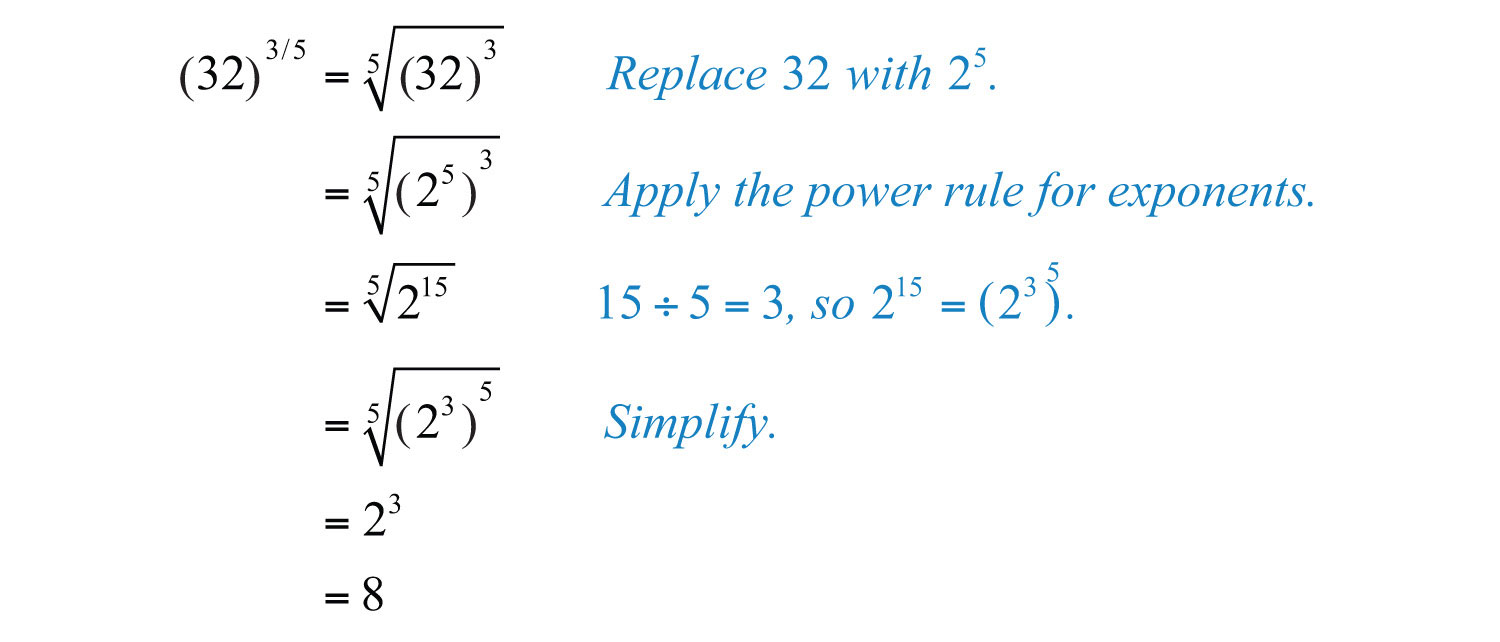Given a radical expression, we will be asked to find the equivalent in exponential form. Assume all variables are positive.

Example 6: Rewrite using rational exponents: $x23$.

Solution: Here the index is 3 and the power is 2. We can writeAnswer: $x2/3$

Example 7: Rewrite using rational exponents: $y36$.

Solution: Here the index is 6 and the power is 3. We can writeAnswer: $y1/2$

It is important to note that the following are equivalent.In other words, it does not matter if we apply the power first or the root first. For example, we can apply the power before the root:Or we can apply the nth root before the power:The results are the same.

Example 8: Rewrite as a radical and then simplify: $(−8)2/3$.

Solution: Here the index is 3 and the power is 2. We can writeTry this! Rewrite as a radical and then simplify: $253/2$.

### Video Solution

(click to see video)

Some calculators have a caret button $^$. If so, we can calculate approximations for radicals using it and rational exponents. For example, to calculate $2=21/2=2^(1/2)≈1.414$, we would typeTo calculate $223=22/3=2^(2/3)≈1.587$, we would type## Operations Using the Rules of Exponents

In this section, we review all of the rules of exponents, which extend to include rational exponents. If given any rational numbers m and n, then we have

 Product rule: $xm⋅xn=xm+n$ Quotient rule: $xmxn=xm−n , x≠0$ Power rule: $(xm)n=xm⋅n$ Power rule for a product: $(xy)n=xnyn$ Power rule for a quotient: $(xy)n=xnyn , y≠0$ Negative exponents: $x−n=1xn$ Zero exponent: $x0=1, x≠0$

These rules allow us to perform operations with rational exponents.

Example 9: Simplify: $22/3⋅21/6$.

Solution: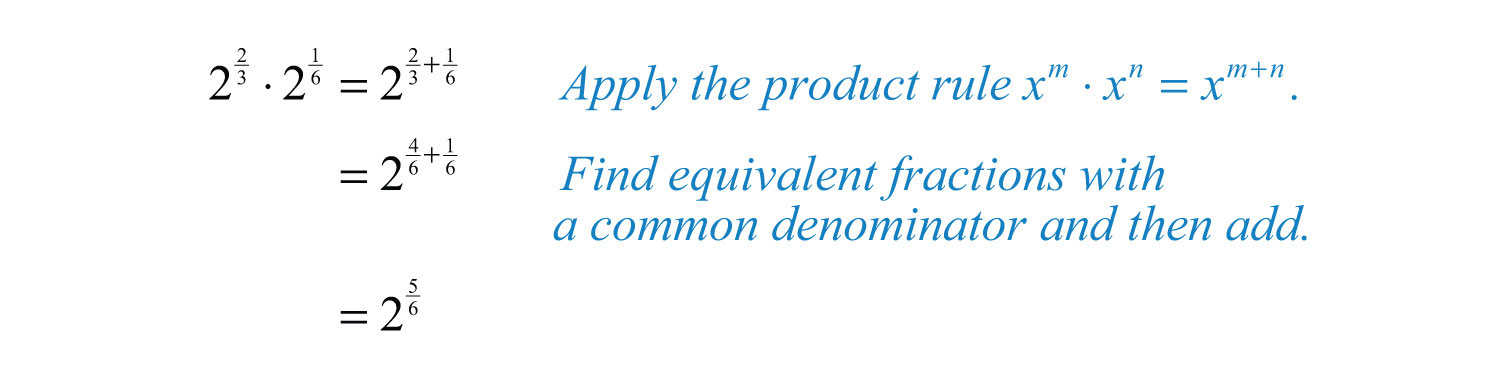Answer: $25/6$

Example 10: Simplify: $x1/2x1/3$.

Solution: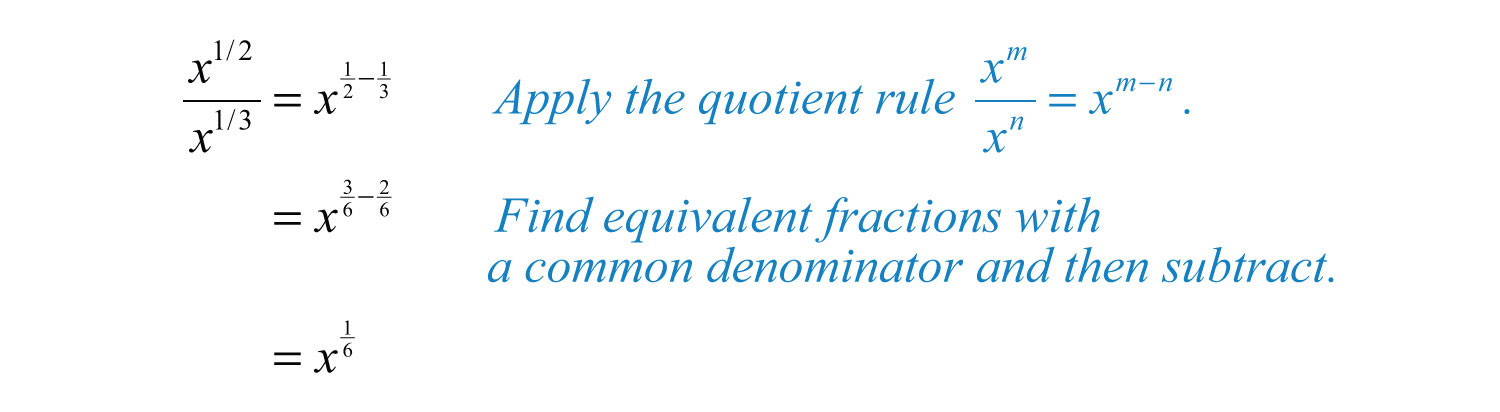Answer: $x1/6$

Example 11: Simplify: $(y3/4)2/3$.

Solution: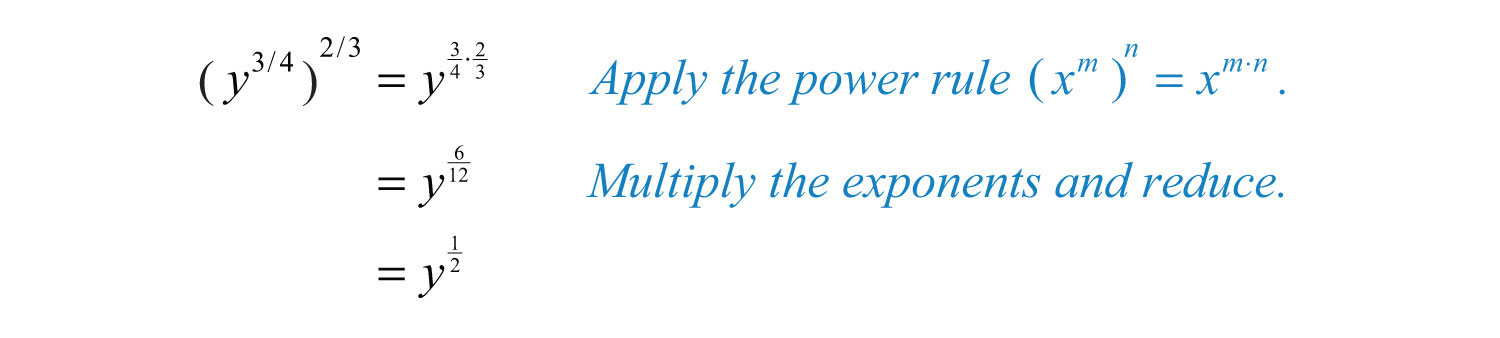Answer: $y1/2$

Example 12: Simplify: $(16a4b8)3/4$.

Solution: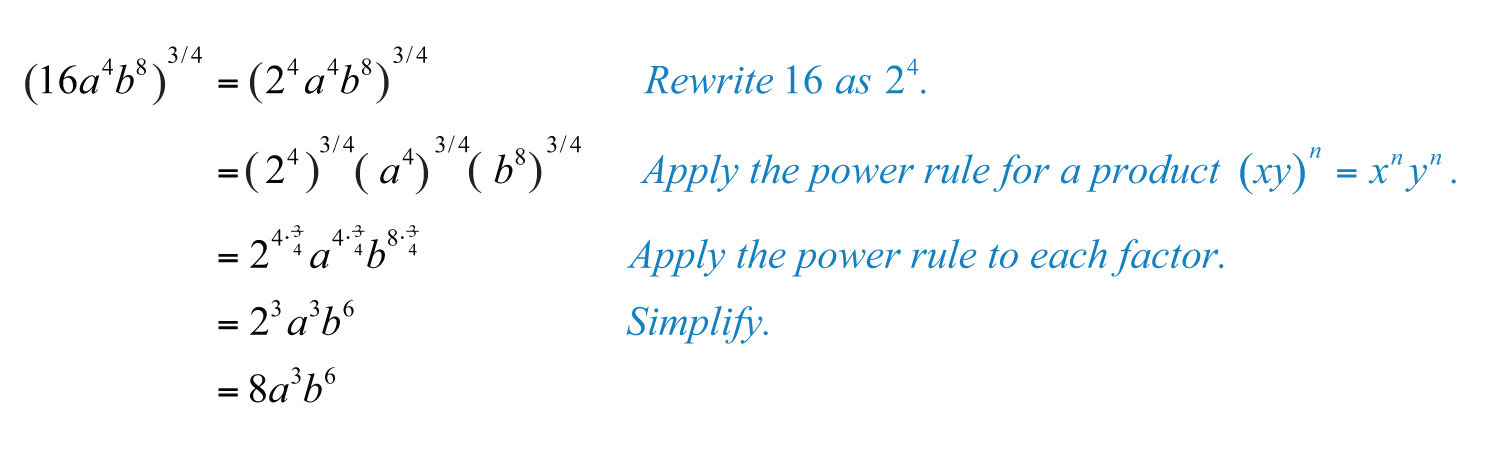Answer: $8a3b6$

Example 13: Simplify: $25−3/2$.

Solution: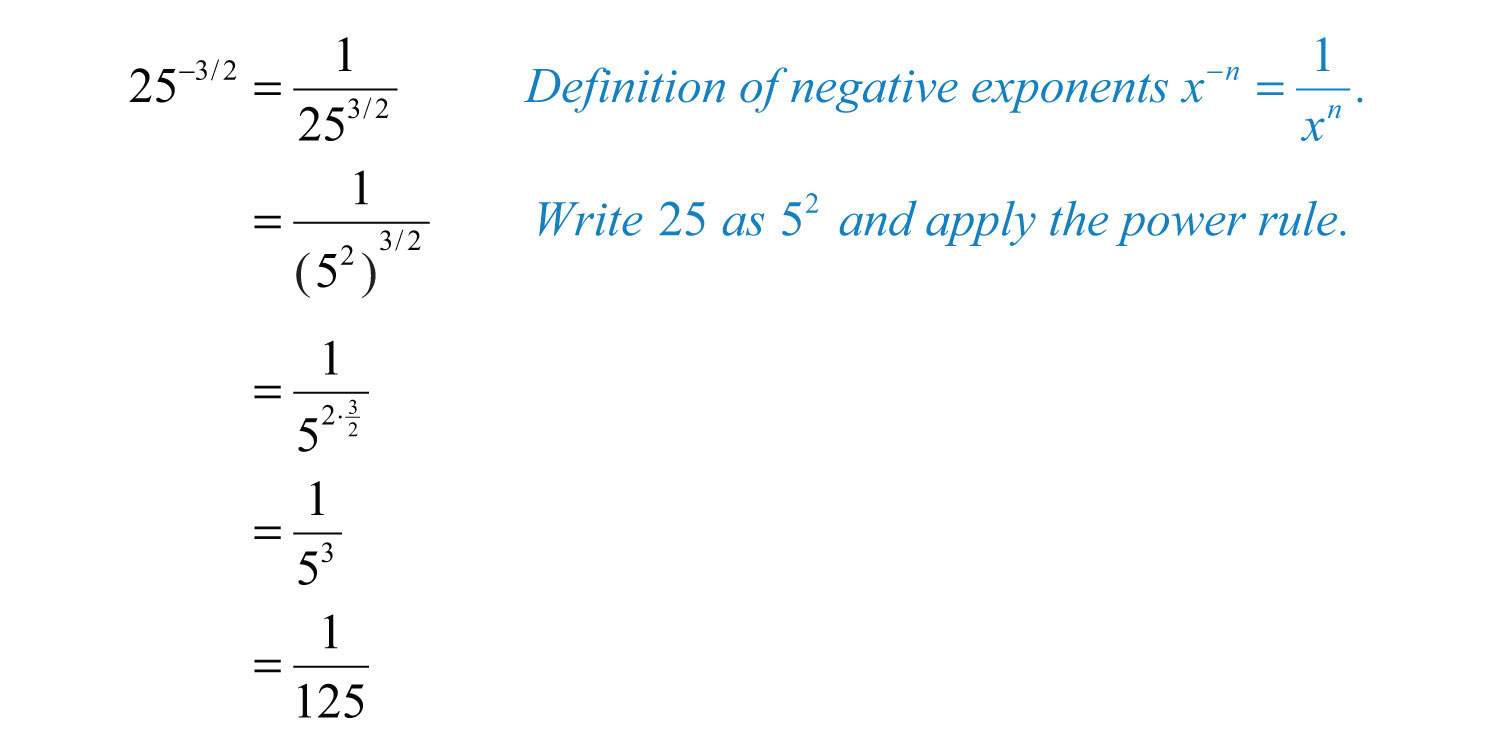Try this! Simplify: $(8 a 3/4 b 3)2/3a1/3$.

Answer: $4a1/6b2$

### Video Solution

(click to see video)

## Radical Expressions with Different Indices

To apply the product or quotient rule for radicals, the indices of the radicals involved must be the same. If the indices are different, then first rewrite the radicals in exponential form and then apply the rules for exponents.

Example 14: Multiply: $2⋅23$.

Solution: In this example, the index of each radical factor is different. Hence the product rule for radicals does not apply. Begin by converting the radicals into an equivalent form using rational exponents. Then apply the product rule for exponents.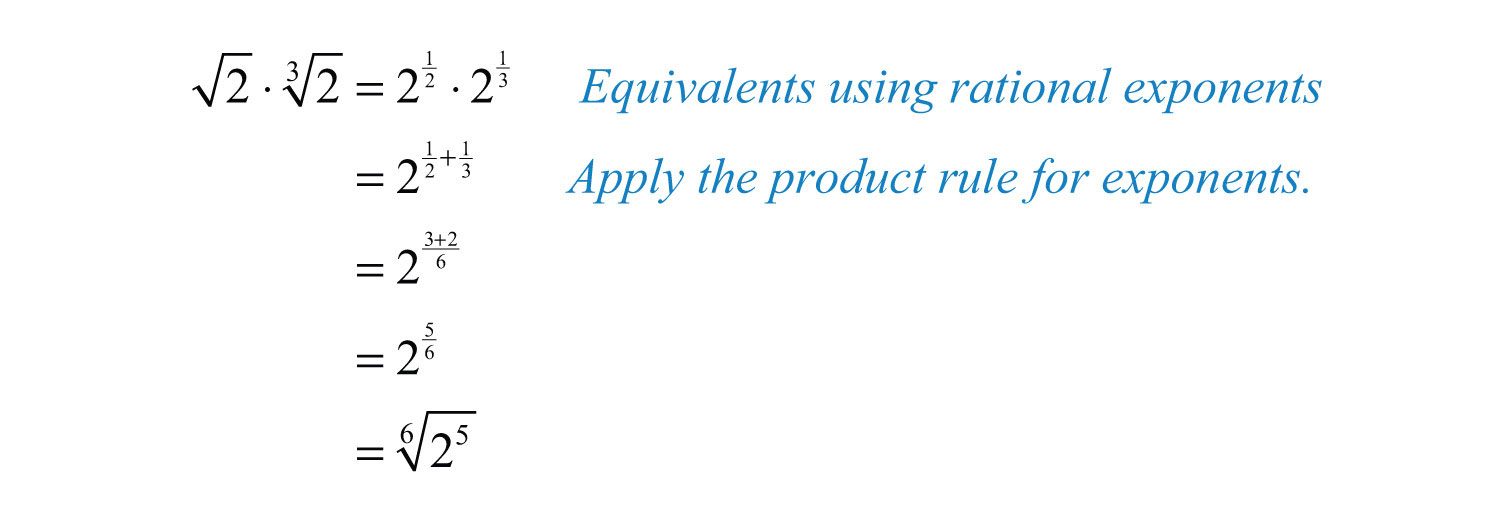Answer: $256$

Example 15: Divide: $4325$.

Solution: In this example, the index of the radical in the numerator is different from the index of the radical in the denominator. Hence the quotient rule for radicals does not apply. Begin by converting the radicals into an equivalent form using rational exponents and then apply the quotient rule for exponents.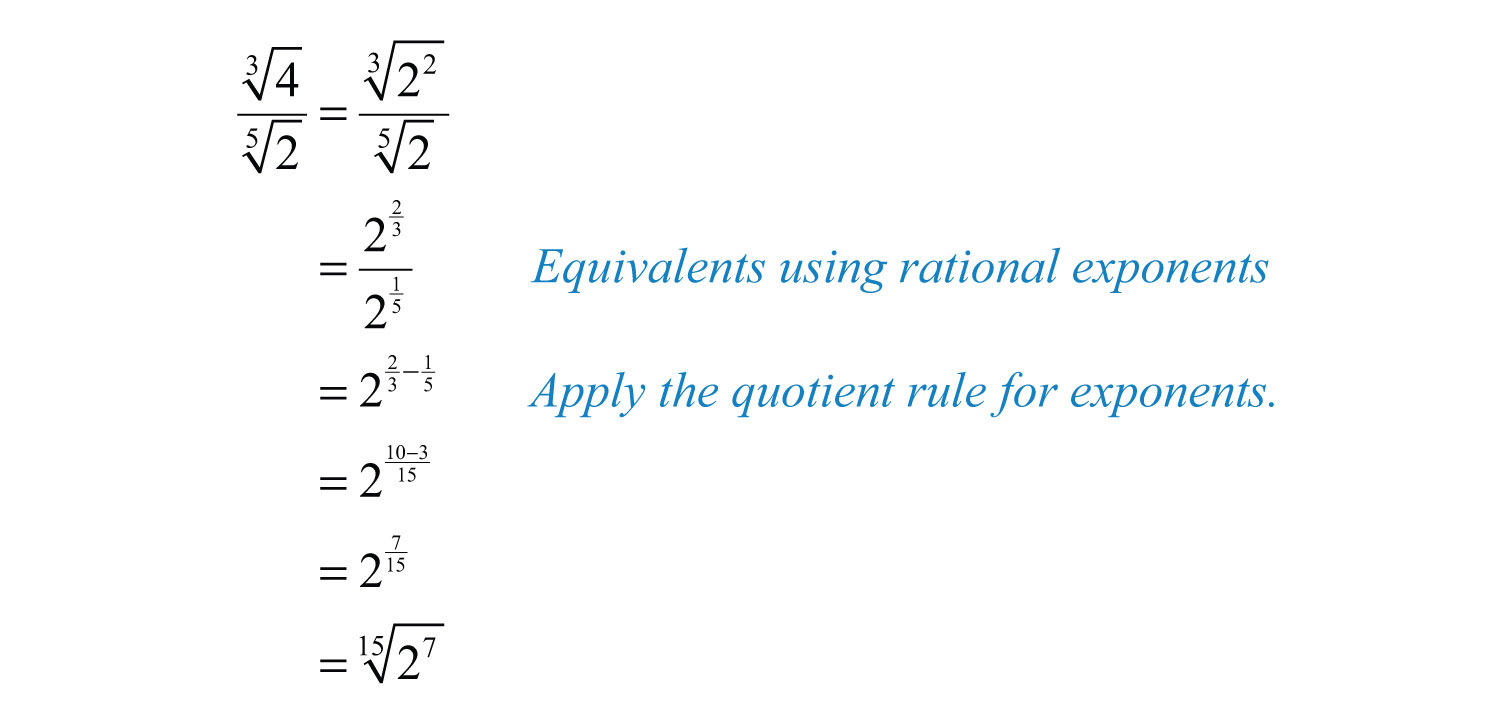Answer: $2715$

Example 16: Simplify: $43$.

Solution: Here the radicand of the square root is a cube root. After rewriting this expression using rational exponents, we will see that the power rule for exponents applies.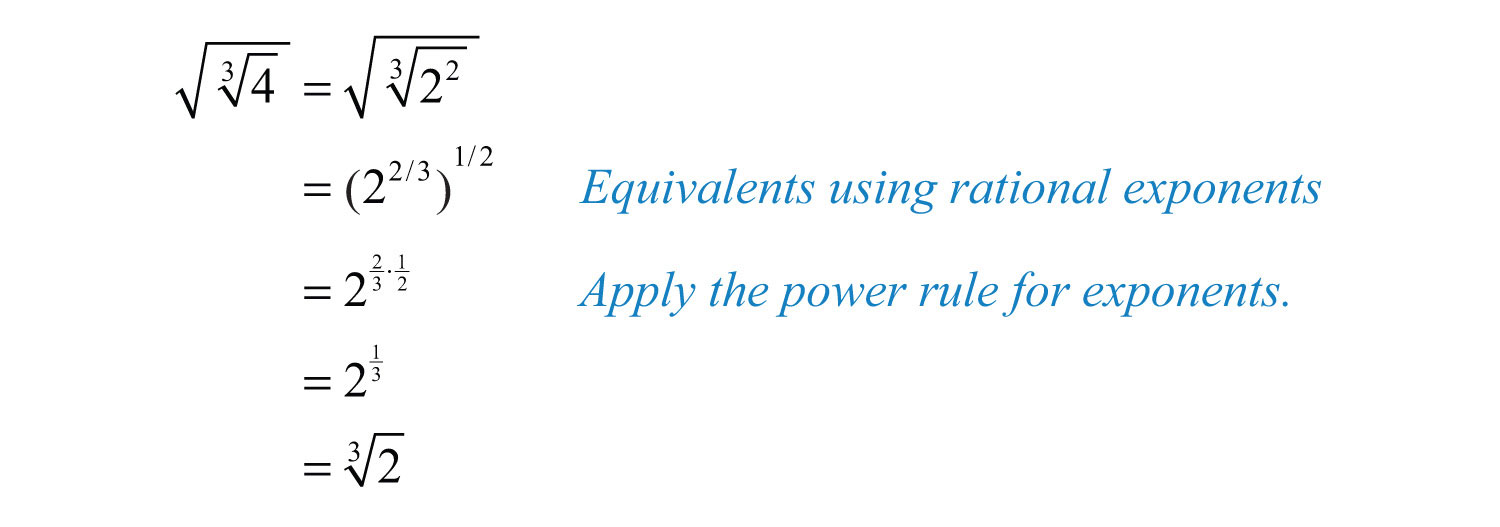Answer: $23$

### Key Takeaways

• When converting fractional exponents to radicals, use the numerator as the power and the denominator as the index of the radical.
• All the rules of exponents apply to expressions with rational exponents.

### Topic Exercises

Part A: Rational Exponents

Express using rational exponents.

1. $6$

2. $10$

3. $113$

4. $24$

5. $523$

6. $234$

7. $x5$

8. $x6$

9. $x76$

10. $x45$

11. $21/2$

12. $51/3$

13. $72/3$

14. $23/5$

15. $x3/4$

16. $x5/6$

17. $x−1/2$

18. $x−3/4$

19. $(1x)−1/3$

20. $(1x)−3/5$

Write as a radical and then simplify.

21. $251/2$

22. $361/2$

23. $1211/2$

24. $1441/2$

25. $(14)1/2$

26. $(49)1/2$

27. $4−1/2$

28. $9−1/2$

29. $(14)−1/2$

30. $(116)−1/2$

31. $81/3$

32. $1251/3$

33. $(127)1/3$

34. $(8125)1/3$

35. $(−27)1/3$

36. $(−64)1/3$

37. $161/4$

38. $6251/4$

39. $81−1/4$

40. $16−1/4$

41. $100,0001/5$

42. $(−32)1/5$

43. $(132)1/5$

44. $(1243)1/5$

45. $93/2$

46. $43/2$

47. $85/3$

48. $272/3$

49. $163/2$

50. $322/5$

51. $(116)3/4$

52. $(181)3/4$

53. $(−27)2/3$

54. $(−27)4/3$

55. $(−32)3/5$

56. $(−32)4/5$

Use a calculator to approximate an answer rounded to the nearest hundredth.

57. $23/4$

58. $32/3$

59. $51/5$

60. $71/7$

61. $(−9)3/2$

62. $−93/2$

63. Explain why (−4)^(3/2) gives an error on a calculator and −4^(3/2) gives an answer of −8.

64. Marcy received a text message from Mark asking her how old she was. In response, Marcy texted back “125^(2/3) years old.” Help Mark determine how old Marcy is.

Part B: Rational Exponents

Perform the operations and simplify. Leave answers in exponential form.

65. $22/3⋅24/3$

66. $33/2⋅31/2$

67. $51/2⋅51/3$

68. $21/6⋅23/4$

69. $y1/4⋅y2/5$

70. $x1/2⋅x1/4$

71. $57/351/3$

72. $29/221/2$

73. $2a2/3a1/6$

74. $3b1/2b1/3$

75. $(81/2)2/3$

76. $(36)2/3$

77. $(x2/3)1/2$

78. $(y3/4)4/5$

79. $(4x2y4)1/2$

80. $(9x6y2)1/2$

81. $(2x1/3y2/3)3$

82. $(8x3/2y1/2)2$

83. $( a 3/4 a 1/2)4/3$

84. $( b 4/5 b 1/10)10/3$

85. $(4 x 2/3 y 4)1/2$

86. $(27 x 3/4 y 9)1/3$

87. $y1/2⋅y2/3y1/6$

88. $x2/5⋅x1/2x1/10$

89. $xyx1/2y1/3$

90. $x5/4yxy2/5$

91. $49a5/7b3/27a3/7b1/4$

92. $16a5/6b5/48a1/2b2/3$

93. $(9 x 2/3 y 6)3/2x1/2y$

94. $(125 x 3 y 3/5)2/3xy1/3$

95. $(27 a 1/4 b 3/2)2/3a1/6b1/2$

96. $(25 a 2/3 b 4/3)3/2a1/6b1/3$

Part C: Mixed Indices

Perform the operations.

97. $93⋅35$

98. $5⋅255$

99. $x⋅x3$

100. $y⋅y4$

101. $x23⋅x4$

102. $x35⋅x3$

103. $100310$

104. $16543$

105. $a23a$

106. $b45b3$

107. $x23x35$

108. $x34x23$

109. $165$

110. $93$

111. $253$

112. $553$

113. $73$

114. $33$

Part D: Discussion Board

115. Who is credited for devising the notation for rational exponents? What are some of his other accomplishments?

116. When using text, it is best to communicate nth roots using rational exponents. Give an example.

1: $61/2$

3: $111/3$

5: $52/3$

7: $x1/5$

9: $x7/6$

11: $2$

13: $723$

15: $x34$

17: $1x$

19: $x3$

21: 5

23: 11

25: 1/2

27: 1/2

29: 2

31: 2

33: 1/3

35: −3

37: 2

39: 1/3

41: 10

43: 1/2

45: 27

47: 32

49: 64

51: 1/8

53: 9

55: −8

57: 1.68

59: 1.38

61: Not a real number

63: In the first expression, the square root of a negative number creates an error condition on the calculator. The square root of a negative number is not real. In the second expression, because of the order of operations, the negative sign is applied to the answer after 4 is raised to the (3/2) power.

65: 4

67: $55/6$

69: $y13/20$

71: 25

73: $2a1/2$

75: 2

77: $x1/3$

79: $2xy2$

81: $8xy2$

83: $a1/3$

85: $2x1/3y2$

87: $y$

89: $x1/2y2/3$

91: $7x2/7y5/4$

93: $27x1/2y8$

95: $9b1/2$

97: $31315$

99: $x56$

101: $x1112$

103: $106$

105: $a6$

107: $x15$

109: $45$

111: $215$

113: $76$